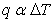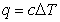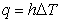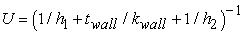﻿ Why Conventional Engineering Science should be Abandoned, and the New Engineering Science that should Replace ItPublications are Open
Access in this journal
Article Versions
Export Article
• Normal Style
• MLA Style
• APA Style
• Chicago Style
Research Article
Open Access Peer-reviewed

### Why Conventional Engineering Science should be Abandoned, and the New Engineering Science that should Replace It

American Journal of Mechanical Engineering. 2021, 9(1), 1-6. DOI: 10.12691/ajme-9-1-1
Received November 21, 2020; Revised December 22, 2020; Accepted December 30, 2020

### Abstract

Conventional engineering science should be abandoned because: Engineering laws that are proportional equations (such as q = hΔT) cannot describe nonlinear phenomena (such as boiling heat transfer). Engineering laws were created by assigning dimensions to numbers, in violation of the conventional view that dimensions must not be assigned to numbers. Contrived parameters (such as heat transfer coefficient) make it impossible to solve nonlinear problems with the variables separated, greatly complicating solutions. All engineering equations are irrational because they attempt to describe how the numerical values and dimensions of parameters are related, when in fact equations can rationally describe only how numerical values are related. In the new engineering science described herein: Engineering laws describe proportional, linear, and nonlinear phenomena. No engineering laws were created by assigning dimensions to numbers. There are no contrived parameters (such as heat transfer coefficient), and therefore nonlinear problems are solved with the variables separated. All engineering equations are rational because they describe only how the numerical values of parameters are related.

### 1. The Genesis of Conventional Engineering Science

Conventional engineering science is largely Fourier’s brainchild 1. Until the publication of his treatise, The Analytical Theory of Heat, in 1822:

• There were no engineering laws in the form of dimensionally homogeneous equations.

• The concept of “flux” had not yet been conceived.

• It had not yet been determined how to solve boundary condition problems.

• In the view of dimensional homogeneity that prevailed at the beginning of the nineteenth century, it was irrational to multiply or divide engineering parameters. That is why Hooke’s law is a proportion rather than an equation, and why Newton’s second law of motion in his treatise The Principia 2 published in 1726 is a proportion rather than an equation.

Fourier’s revolutionary view of dimensional homogeneity made it possible, for the first time in the history of science, to rationally multiply and divide engineering parameters, thereby making it possible to conceive dimensionally homogeneous equations that describe how engineering parameters are related.

Fourier conceived the first equations/laws of conventional engineering science, the law of convection heat transfer and the law of conduction heat transfer. Fourier’s revolutionary view of dimensional homogeneity, and other engineering methodologies he conceived, enabled others to conceive and apply many of the laws of conventional engineering science.

### 2. What Eq. (1) Means

Equation (1) is the law of convection heat transfer conceived by Fourier (but often erroneously credited to Newton).(1)

Based on conventional symbolism, Eq. (1) means:

q is always proportional to ΔT.

h is always a symbol for q/ΔT.

q = hΔT (q/ΔT)ΔT

h (ie q/ΔT) is always independent of ΔT.

h (ie q/ΔT) is always a constant.

• Eq. (1) is always dimensionally homogeneous because q is always proportional to ΔT.

### 3. Why Eq. (1) does notGenerically Describe q{ΔT} or h, and Therefore cannot be a Law

In conventional engineering science, Eq. (1) is generally said to be a law. If Eq. (1) were in fact a law, q would always be proportional to ΔT, and h would always be a symbol for q/ΔT.

It has been known for more than a century that, if heat transfer is by natural convection, condensation, or boiling, q is a nonlinear function of ΔT. Therefore:

• Equation (1) cannot be a law because it does not generically describe convection heat transfer. It can describe proportional phenomena (such as forced convection heat transfer to a one phase fluid) because it is a proportional equation, but it cannot describe nonlinear phenomena (such as boiling heat transfer) because it is a proportional equation.

• Equation (1) does not generically describe h because h is a constant if q is proportional to ΔT, and h is a variable if q is not proportional to ΔT.

### 4. Why Eq. (2) should be the Law of Convection Heat Transfer in Conventional Engineering Science

Equation (2) should be the law of convection heat transfer in conventional engineering science because, in conventional engineering science, it would be a rigorously correct and dimensionally homogeneous equation that generically describes q{ΔT} and h.(2)

Equation (2) states that q{ΔT} may be proportional, or linear, or nonlinear. And h always equals q/f{ΔT}. If f{ΔT} equals ΔT, h equals q/ΔT. If f{ΔT} does not equal ΔT, h equals q/f{ΔT}.

### 5. How Fourier Created q =hΔT by Assigning Dimensions to a Number

Until the nineteenth century, there were no equations/laws that describe how stress is related to strain, or how convection heat flux is related to boundary layer temperature difference. Until the nineteenth century, the prevailing view of dimensional homogeneity considered it irrational to multiply or divide parameters, thereby making it impossible to conceive dimensionally homogeneous equations/laws that describe how engineering parameters are related.

Fourier performed experiments in forced convection heat transfer to atmospheric air, and concluded that, if heat transfer is by steady-state forced convection to atmospheric air, heat flux q is always proportional to temperature difference ΔT, as in Proportion (3).(3)

Proportion (3) would have satisfied Hooke and Newton, but it did not satisfy Fourier. He wanted an equation, and it had to be dimensionally homogeneous. When Proportion (3) is transformed to an equation, Eq. (4) results in which c is a number.(4)

Fourier 3 recognized that Eq. (4) is unacceptable because it is not dimensionally homogeneous. He realized that Eq. (4) could be transformed to a homogeneous equation only if dimensions could rationally be assigned to numbers, and parameters could rationally be multiplied and divided. Consequently he conceived a revolutionary view of dimensional homogeneity in which it is rational to assign dimensions to numbers, and rational to multiply and divide parameters.

Fourier’s revolutionary view of dimensional homogeneity made it possible to conceive dimensionally homogeneous equations that describe how engineering parameters are related. Using his view of dimensional homogeneity, and other engineering methodologies he conceived, Fourier conceived, and explained how to apply, the heat transfer laws of convection and conduction, the first laws of conventional engineering science.

Fourier described his revolutionary view of dimensional homogeneity in the following: 4

. . . every undetermined magnitude or constant has one dimension proper to itself, and the terms of one and the same equation could not be compared, if they had not the same exponent of dimension . . .this consideration is derived from primary notions on quantities; for which reason, in geometry and mechanics, it is the equivalent of the fundamental lemmas which the Greeks have left us without proof.

In his nearly 500 page treatise, The Analytical Theory of Heat published in 1822, Fourier:

• Made no effort to prove the validity of his revolutionary view of dimensional homogeneity.

• Did not include the fundamental lemmas in his treatise.

• Did not cite a reference that included the fundamental lemmas.

It has never been proven that Fourier’s view of dimensional homogeneity is rational. Presumably, his colleagues accepted his unproven view because it enabled him to solve problems they were unable to solve.

Fourier’s description of his view of dimensional homogeneity includes the statement “every . . . constant has one dimension proper to itself”. In other words, Fourier states that numbers in equations inherently have dimension, and therefore it is rational to assign dimensions to numbers in equations.

To number c in Eq. (4), Fourier assigned the symbol h and the dimensions that made Eq. (4) homogeneous. That is how Fourier created h, and how he transformed dimensionally inhomogeneous Eq. (4) to dimensionally homogeneous Eq. (1).(1)

### 6. How Fourier Defined h, and what Eq. (1) and h Meant in the Nineteenth Century

Fourier defined h in the following: 5

We have taken as the measure of the external conducibility of a solid body a coefficient h, which denotes the quantity of heat which would pass, in a definite time (a minute), from the surface of this body, into atmospheric air, supposing that the surface had a definite extent (a square metre), that the constant temperature of the body was 1, and that of the air 0, and that the heated surface was exposed to a current of air of a given invariable velocity.

Fourier defined h to be a proportionality constant and the symbol for q/ΔT. Fourier’s definition requires that the heat transfer fluid be atmospheric air, and that there be a steady-state current of air over the heat transfer surface. In other words, Eq. (1) applies only if the heat transfer fluid is atmospheric air, and there is a steady-state current of air over the heat transfer surface.

In the nineteenth century, Eq. (1) was not just an equation. It was a law, and the law meant that, if the heat transfer fluid is atmospheric air, and if there is a steady-state current of air over the heat transfer surface, q is always proportional to ΔT, h is always independent of ΔT, h is always a proportionality constant, and h is always a symbol for q/ΔT.

### 7. Why Laws such as q = hΔT are irrational

The coefficient in q = hΔT, and coefficients in other laws that are proportional equations, were created by assigning dimensions to numbers. In Fourier’s view of dimensional homogeneity, it was rational to assign dimensions to numbers in equations.

Although Fourier is generally credited with the conventional view of dimensional homogeneity, the conventional view differs from Fourier’s view in one important way. Langhaar 6 states:

Dimensions must not be assigned to numbers, for then any equation could be regarded as dimensionally homogeneous.

Therefore q = hΔT and h, and other laws in the form of proportional equations and their coefficients, are irrational because they were created by assigning dimensions to numbers, in violation of the conventional view that dimensions must not be assigned to numbers.

If laws such as q = hΔT are not abandoned, the conventional view of dimensional homogeneity must be revised in order to again make it rational to assign dimensions to numbers.

### 8. Maxwell’s (1831–1879) View of Ohm’s Law and Electrical Resistance R, and what He Would Have Recommended if He Had Known that h is not always Independent of ΔT

In A Treatise on Electricity and Magnetism 7 published in 1873, Clerk Maxwell explained why Ohm’s Law{1}, Eq. (5), was a true law, and why R had scientific value.(5)

Maxwell wrote:

. . .the Resistance of a conductor . . . is defined to be the ratio of the electromotive force to the strength of the current which it produces . . .

. . . the Resistance of a conductor . . .would have been of no scientific value unless Ohm had shewn, as he did experimentally, . . . (that) the resistance of a conductor is independent of the strength of the current flowing through it.

The resistance of a conductor may be measured to within one ten thousandth or even one hundred thousandth part of its value, and so many conductors have been tested that our assurance of the truth of Ohm’s Law is now very high.

In 1873, Ohm’s law was a true law only because it applied to all known conductors, and electrical resistance had scientific value only because the resistance of all known conductors was “independent of the strength of the current flowing through it”.

Maxwell’s words make it certain that, had he lived until semiconductors were invented, he would surely have said that Ohm’s law is no longer a true law because it is not always obeyed, and R no longer has scientific value because it is not always independent of I. And Maxwell would surely have recommended that Ohm’s law and R be abandoned.

Maxwell’s words also make it certain that, had he known that h is not always independent of ΔT, he would surely have concluded that q = hΔT is no longer a true law because it is not always obeyed, and h no longer has scientific value because it is not always independent of ΔT. And Maxwell would surely have recommended that q = hΔT and h be abandoned.

### 9. Engineering Science in the Twentieth Century, Why Fourier’s Law of Convection Heat Transfer had to be abandoned or revised, and the de facto Law of Convection Heat Transfer Used in Conventional Engineering Science

Sometime near the beginning of the twentieth century, it was realized that, if heat transfer is by natural convection, condensation, or boiling, q is a nonlinear function of ΔT, and Eq. (1) does not apply because it is a proportional equation. Consequently it became necessary to decide what equation would replace Eq. (1). The viable alternatives were:

• Abandon Eq. (1), and conceive a new law of convection heat transfer that generically describes q{ΔT}.(1)

• Replace Eq. (1) with Eq. (2). Equation (2) is always dimensionally homogeneous. It states that q is always a function of ΔT, the function may be proportional, linear, or nonlinear, and h is always a symbol for the variable q/f{ΔT}.(2)

• Describe Eq. (1) as the law of convection heat transfer, and use Eq. (2) as the de facto law of convection heat transfer.

In conventional engineering science, Eq. (1) is described as the law of convection heat transfer, and Eq. (2) is the de facto law of convection heat transfer.

The proof that Eq. (2) is the de facto law of convection heat transfer lies in the fact that many h correlations in the literature indicate that h is a variable dependent on ΔT (as in Eq. (2)), and not a constant independent of ΔT (as in Eq. (1)).

### 10. How Contrived Parameters such as h (ie q/f{ΔT}) Greatly Complicate the Solution of Problems that Concern Nonlinear Behavior

One of the first lessons in mathematics is that it is desirable to separate the variables in nonlinear equations because the solution of nonlinear equations is much simpler if the variables are separated. If a contrived parameter such as h is used in a nonlinear equation, it is impossible to separate the variables q and ΔT because h is a symbol for q/f{ΔT}.

If q and ΔT are not separated, h (ie q/f{ΔT}) is an extraneous variable in nonlinear equations, and it greatly complicates their solution because equations in three variables (q, q/f{ΔT}, and ΔT) are much more difficult to solve than equations in two variables (q and ΔT).

### 11. Why All Engineering Proportions in Conventional Engineering Science Are Irrational

• Pigs cannot be proportional to trees because pigs and trees are different things, and different things cannot be proportional.

• Worms cannot be proportional to airplanes because worms and airplanes are different things, and different things cannot be proportional.

• Stress cannot be proportional to strain because stress and strain are different things, and different things cannot be proportional.

All engineering proportions in conventional engineering science are irrational because they state that the numerical values and dimensions of parameters are proportional, whereas they can rationally state only that the numerical values of different things are proportional.

In the new engineering science, all proportions state that numerical values are proportional. For example, Hooke’s law is the numerical value of stress is proportional to the numerical value of strain. Therefore, if Hooke’s law is described symbolically, the symbols must represent numerical value, but not dimension.

It has long been assumed that, although equations must be dimensionally homogeneous, proportions need not be dimensionally homogeneous. Because a proportion that relates σ and ε is identical to a proportional equation that qualitatively relates σ and ε, proportions should be dimensionally homogeneous. In the new engineering science, all proportions are dimensionally homogeneous.

### 12. Why All engineering Equations in Conventional Engineering Science Are Irrational

• Equations cannot describe how pigs and trees are related because pigs and trees are different things, and different things cannot be related.

• Equations cannot describe how worms and airplanes are related because worms and airplanes are different things, and different things cannot be related.

• Equations cannot describe how stress and strain are related because stress and strain are different things, and different things cannot be related.

• Equations cannot describe how different things are related because different things cannot be related.

• Equations can describe only how the numerical values of parameters are related.

In conventional engineering science, all engineering equations are irrational because they all attempt to describe how numerical values and dimensions of parameters are related, when in fact they can only describe how numerical values are related.

In the new engineering science, parameter symbols in all equations represent numerical value, but not dimension. Consequently all engineering equations are rational because they all describe only how the numerical values of parameters are related, and therefore they all are inherently dimensionless and dimensionally homogeneous. (If an equation is quantitative, the dimension units that underlie parameter symbols must be specified in an accompanying nomenclature.)

### 13. Why It Is Important to Know that, in the New Engineering Science, Proportions and Equations Are Inherently Dimensionless

In conventional engineering science, parameters such as h and E are required for only one reason: so that engineering laws in the form of proportional equations (such as q = hΔT) can be dimensionally homogeneous. In the new engineering science, equations are inherently dimensionally homogeneous because parameter symbols in equations represent only numerical value.

It is important to know that, in the new engineering science, proportions and equations are inherently dimensionless and dimensionally homogeneous because it means that contrived parameters such as h and E are not required for dimensional homogeneity, and can be abandoned.

### 14. The New Engineering Science

In the new engineering science:

• Engineering laws are analogs of y = f{x}.

• Parameter symbols in equations represent numerical values, but not dimensions. If an equation is quantitative, the dimension units that underlie parameter symbols must be specified in an accompanying nomenclature.

All parametric equations are inherently dimensionless and dimensionally homogeneous because all parameter symbols in equations represent numerical value, but not dimension.

No parameters are created by assigning dimensions to numbers. In other words, there are no contrived parameters such as E, h, U, R, etc.

All problems are solved with the primary parameters separated because there are no contrived parameters (such as h (ie q/f{ΔT}) that combine them.

### 15. How to Transform Textbooks from Conventional to New Engineering Science

In order to transform heat transfer textbooks from conventional to new engineering science:

• Revise the nomenclature by removing U, h, and k. Include the statements: All parameter symbols represent numerical value but not dimension. If a parameter symbol is used in a quantitative equation, the dimension units that underlie the symbol are specified in the nomenclature.

• Replace q = hΔT with q = f{ΔT}.

• Transform h{ΔT} equations to ΔT{q} equations by substituting q/ΔT for h in all equations that explicitly or implicitly include h, then separating q and ΔT. (Substitute q/ΔT rather than q/f{ΔT} because in conventional engineering science, h is a symbol for q/ΔT.)

• Replace Eq. (6) with Eq. (7). Note that Eq. (7) is intuitively simple, whereas Eq. (6) is so complex it requires proof of validity. (To prove that Eqs. (6) and (7) are identical, substitute q/ΔT for U, h1, h2, and k/t, then separate q and ΔT.)(6)(7)

Equation (6) may have one, two, or three unknown variables (because h1 and h2 may be variables), whereas Eq. (7) has only one unknown variable. If Eq. (6) has two or three unknown variables, it is much more difficult to solve than Eq. (7).

Textbooks for other branches of engineering science are transformed in a similar way.

### 16. Conclusions

The new engineering science should replace conventional engineering science because it:

• Is much more rational because there are no contrived parameters such as h, and consequently real engineering phenomena (such as heat transfer) are always described by equations that contain only real parameters (such as q and ΔT).

• Makes it much easier to think about and understand engineering phenomena and engineering problems because there are only real parameters to think about and understand.

• Is much more rational because engineering equations describe how the numerical values of parameters are related, and not how the numerical values and dimensions of parameters are related.

• Makes it much easier to learn engineering science (as demonstrated by Eqs. (6) and (7)), because it reduces the number of parameters that must be understood and applied.

• Makes it easier to learn engineering science because the abandonment of contrived parameters means that it is not necessary to learn how to solve nonlinear equations without separating the variables. (Solving nonlinear equations without separating the variables is not something generally taught in courses on mathematics.)

• Greatly simplifies the solution of problems that concern nonlinear behavior because the abandonment of contrived parameters reduces the number of variables in problems that concern nonlinear behavior.

### Nomenclature

Note: Symbols may represent numerical value and dimension, or numerical value alone.

a acceleration

c arbitrary constant

f force

h symbol for q/f{ΔT}

q heat flux

T temperature

ε strain

σ stress

### Note

1. Eq. (5) is not Ohm’s law by Ohm. Ohm’s law by Ohm is E = IL in which L is the length of a copper wire of a standard diameter. Although E = IL is not dimensionally homogeneous, it prevailed for 30 or 40 years. It seems likely that Ohm was familiar with Fourier’s view of dimensional homogeneity, and that he did not agree that rational equations are necessarily dimensionally homogeneous. From a practical standpoint, E = IL is preferable to E = IR because L is tangible, and R is not—ie preferable because it contains only real parameters.

### References

  Adiutori, E. F., August 2005, “Fourier”, Mechanical Engineering, pp 30-31. In article View Article  Newton, I., The Principia, 1726, 3rd edition, translation by Cohen, I.B. and Whitman, A. M., 1999, p 460, University of California Press. In article  Fourier, J., 1822, The Analytical Theory of Heat, Article 75, 1955 Dover edition of 1878 English translation, The University Press In article  Fourier, J., 1822, The Analytical Theory of Heat, Article 160, 1955 Dover edition of 1878 English translation, The University Press In article  Fourier, J., 1822, The Analytical Theory of Heat, Article 36, 1955 Dover edition of 1878 English translation, The University Press In article  Langhaar, H. L., 1951, Dimensional Analysis and Theory of Models, p. 13, John Wiley & Sons. In article  Maxwell, J. C., 1873, Electricity and Magnetism, pp 295, 296, Macmillan. In articleThis work is licensed under a Creative Commons Attribution 4.0 International License. To view a copy of this license, visit http://creativecommons.org/licenses/by/4.0/